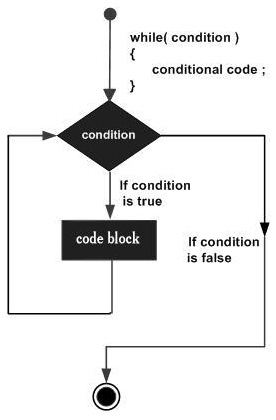# Java while loop

while loop statement in Java programming language repeatedly executes a target statement as long as a given condition is true.

## Syntax

The syntax of a while loop is −

while(Boolean_expression) {
// Statements
}

Here, statement(s) may be a single statement or a block of statements. The condition may be any expression, and true is any non zero value.

When executing, if the boolean_expression result is true, then the actions inside the loop will be executed. This will continue as long as the expression result is true.

When the condition becomes false, program control passes to the line immediately following the loop.

## Flow DiagramHere, the key point of the while loop is that the loop might not ever run. When the expression is tested and the result is false, the loop body will be skipped and the first statement after the while loop will be executed.

### Example

Live Demo

public class Test {

public static void main(String args[]) {
int x = 10;

while( x < 20 ) {
System.out.print("value of x : " + x );
x++;
System.out.print("");
}
}
}

This will produce the following result −

### Output

value of x : 10
value of x : 11
value of x : 12
value of x : 13
value of x : 14
value of x : 15
value of x : 16
value of x : 17
value of x : 18
value of x : 19